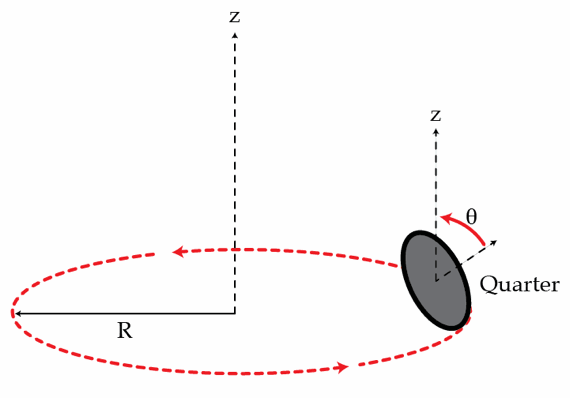# Rolling coin

A U.S quarter is rolling on the floor without slipping in such a way that it describes a circular path of radius $R=4~\mbox{cm}$. The plane of the coin is tilted at an angle of $\theta=45^{\circ}$ with respect to the horizontal plane (see the figure below). Find the coin's period $T$ in seconds, that is, the time it takes for the coin to go around the circle of radius $R$. The radius of a U.S quarter is $r=1.2 ~\mbox{cm}$.Details and assumptions

Assume $g=9.8~\mbox{m/s}^{2}$.

×

Problem Loading...

Note Loading...

Set Loading...